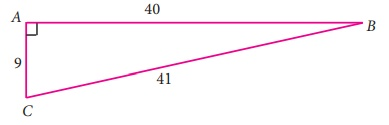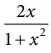Home | | Maths 9th std | Exercise 6.1: Trigonometry Basic

# Exercise 6.1: Trigonometry Basic

Maths : Trigonometry: Book Back, Exercise, Example Numerical Question with Answers, Solution: Exercise 6.1: Trigonometry Basic

Trigonometry: Basic

Exercise 6.1

1. From the given figure, find all the trigonometric ratios of angle B.2. From the given figure, find the values of

(i) sin B (ii) sec B (iii) cot B (iv) cos C (v) tanC (vi) cosecC3. If 2 cos θ = √3 , then find all the trigonometric ratios of angle θ .4. If cos A = 3/5, then find the value of (sin A−cosA) / 2tanA.5. If cos A =, then find the values of sinA and tanA in terms of x.6. If sin θ, then show that bsin θ = acos θ .7. If 3cot A = 2 , then find the value of  [ 4sinA-3cos A ] / [ 2sinA+3cos A ].8. If cos θ : sin θ = 1 : 2, then find the value of [8cosθ-2sinθ ] / [ 4cosθ+2sinθ].9. From the given figure, prove that θ+φ=90º. Also prove that there are two other right angled triangles. Find sinα, cosβ and tanϕ.10. A boy standing at a point O finds his kite flying at a point P with distance OP=25m. It is at a height of 5m from the ground. When the thread is extended by 10m from P, it reaches a point Q. What will be the height QN of the kite from the ground? (use trigonometric ratios)Tags : Numerical Problems with Answers, Solution | Trigonometry | Maths , 9th Maths : UNIT 6 : Trigonometry
Study Material, Lecturing Notes, Assignment, Reference, Wiki description explanation, brief detail
9th Maths : UNIT 6 : Trigonometry : Exercise 6.1: Trigonometry Basic | Numerical Problems with Answers, Solution | Trigonometry | Maths